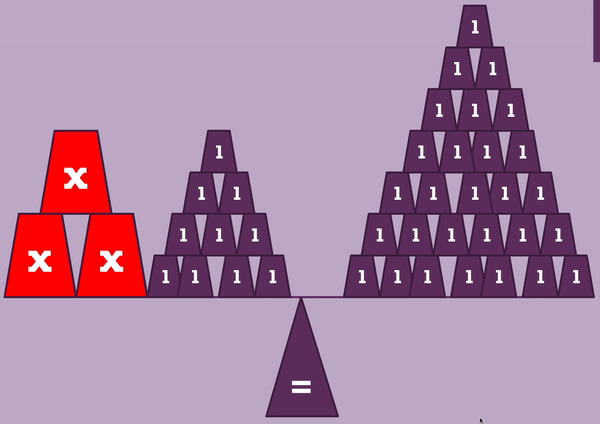Teacher ResourcesPaper Clip World Record

Use Maths skills to get into the Guinness Book of Records

Topics

Rates of Change, Slope, Functions and Graphs, Statistics

Level

JCOL, JCHL

Time

40mins +American Idol

Whatever happened to American Idol? Here we use Statistics to find out.

Topics

Probability, Statistics

Level

JCOL, JCHL, LCOL

Time

30-40minsBalancing Scales, Solving Equations

Using the principles of weighing scales to solve Linear Equations.

Topics

Algebra, Solving Equations, Linear Equations

Level

JCOL, JCHL

Time

30mins3 Shots

A dramatic Basketball match. Use probability to predict will the team win.

Topics

Probability, Experimental Probability, Tree Diagrams

Level

JCOL, JCHL, LCOL, LCHL

Time

30-40minsDueling Discounts

Two coupons. €20 and 20%. Which is more effective?

Topics

Percentages

Level

JCOL, JCHL

Time

15minsDrill Bit Fractions

Using knowledge of fractions to compare different drill bits.

Topics

Fractions

Level

JCOL, JCHL, LCOL

Time

15minsSlope of Hills

Using slope to calculate the gradient of some well known hills.

Topics

Slope, Percentages, Trigonometry, Coordinate Geometry

Level

JCOL, JCHL, LCOL

Time

30-40minsMeatballs

Using volume to solve a food related problem.

Topics

Area, Perimeter and Vol, Volume, Geometry

Level

JCOL, JCHL, LCOL

Time

20-30minsJoulies

Investigating how different Rates of Change are plotted on a graph.

Topics

Functions and Graphs, Coordinate Geometry, Slope, Rates of Change

Level

LCOL, JCHL, JCOL

Time

20-30minsRolling Sellotape

Investigating the connection between a rotating disc, and its circumference.

Topics

Geometry, Area, Perimeter and Vol

Level

LCOL, JCHL, JCOL

Time

30-40minsToothpicks Problem

An introduction exercise to Patterns and Sequences for JC AND LC.

Topics

Patterns and Sequences, Sequences and Series

Level

LCHL, LCOL, JCHL, JCOL

Time

15-20minsRock Paper Scissors

An interesting approach to Experimental Probability and Relative Frequency

Topics

Probability, Statistics, Relative Frequency, Experimental Probability

Level

LCHL, LCOL, JCHL, JCOL

Time

30-40mins